## Excel VDB function

The VBD function calculates the depreciation of an asset for a given time period (including partial periods), using the double-declining balance method or another specified method. VDB stands for variable declining balance.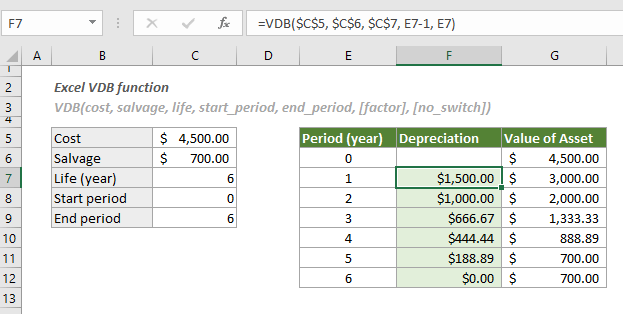#### Syntax

VDB(cost, salvage, life, start_period, end_period, [factor], [no_switch])

#### Arguments

Cost (Required): The initial cost of the asset;
Salvage (Required): The salvage value at the end of the lifetime of the asset. This value can be 0;
Life (Required): The number of periods over which the asset is depreciated;
Start_period (Required): The starting period for which you want to calculate the depreciation;
End_period (Required): The ending period for which you want to calculate the depreciation;
Factor (Optional): The rate at which the balance declines. If it is omitted, it takes on the default value of 2;
No_switch (Optional): A logical value that specifies whether to switch to straight-line depreciation.
When the depreciation is greater than the declining balance calculation, there are two possible values:
-- TRUE: Excel will not switch to the straight-line depreciation method and use the declining balance method of depreciation.
-- FALSE or omitted: Excel will switch to the straight-line depreciation method.

#### Remarks

1. Make sure that the arguments “period” and “life” have the same units of time.
2. You can change the argument “factor” if you do not want to use the double-declining balance method.
3. All arguments except no_switch must be positive numbers.
4. The #VALUE! error occurs when any of the arguments is non-numeric;
5. The #NUM! error occurs when one of the following conditions is met:
-- The “life” is ≤ 0
-- The “start_period” is greater than the “end_period”;
-- if any of the values of the arguments “cost” “salvage”, “start_period”, “end_period” and [factor] is < 0.

#### Return Value

It returns a numeric value.

#### Example

As shown in the screenshot below, the table on the left contains information of an asset, and the table on the right is going to calculate the depreciation and the new value of this asset for each year listed in E6:E11, you can do as follows to get it done.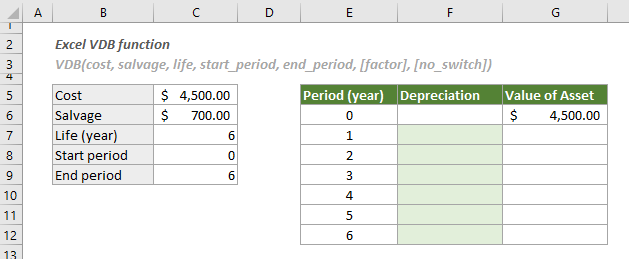1. Select a blank cell (here I select cell F7), copy or enter the formula below and press the Enter key to get the first year’s depreciation. Select this result cell and then drag its AutoFill Handle down to get the depreciation for other years.

=VDB(\$C\$5, \$C\$6, \$C\$7, E7-1, E7)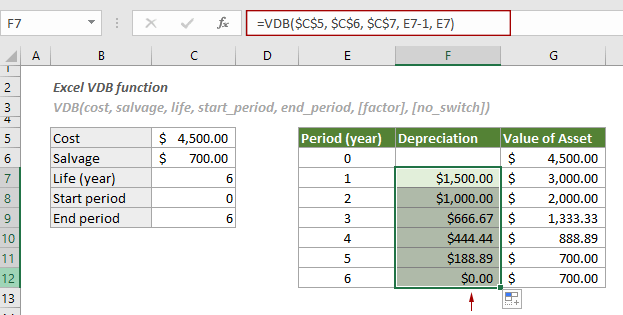Notes:

1) In the formula, the results of “E7-1” and “E7” are “1-1=0” and “1”, which represents the first year period of the asset;
2) In cell G7, we calculate the new value of the asset by subtracting the depreciation from the cost of the asset at the beginning (G6), and then drag its AutoFill Handle down to get the new values of the asset in other years.

=G6-F7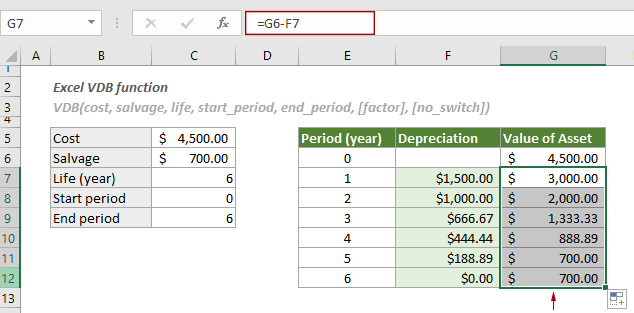3) To calculate the total depreciation of the asset for the whole period (6 years), please apply the following formula.

=VDB(\$C\$5, \$C\$6, \$C\$7, 0, 6)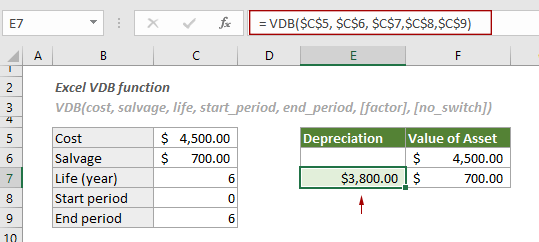4)To calculate the depreciation between two specified periods such as the sixth month and the eighteenth month, apply the formula below:

= VDB(\$C\$5, \$C\$6, C7*12, 6, 18)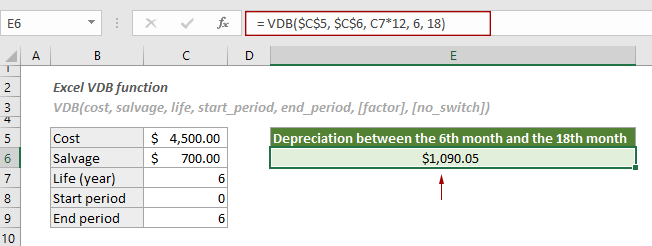5)You can calculate the depreciation between two period using a specified factor instead of the double-declining balance method.

For example, to calculate the depreciation between the sixth month and the eighteenth month using the factor of 1.3, please apply the formula below:

= VDB(\$C\$5, \$C\$6, C7*12, 6, 18, 1.3)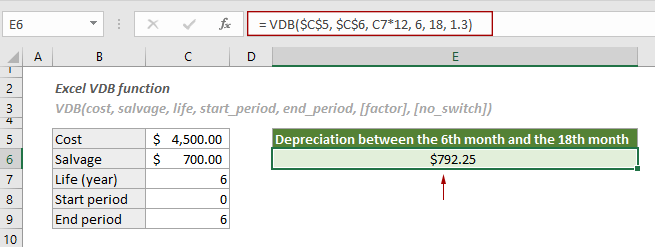#### Related Functions

Excel SLN function
The SLN function calculates the depreciation of an asset for one period based on the straight-line depreciation method.

Excel DDB function
The DDB function returns the depreciation of an asset for a specified period by using a double-declining balance method or other specific method.

Excel DB function
The DB function returns the depreciation of an asset for a specified period by using a fixed-declining balance method.

Excel SYD function
The SYD function calculates the depreciation of an asset for a given period based on the sum-of-years’ digits depreciation method.

### The Best Office Productivity Tools

#### Kutools for Excel - Helps You To Stand Out From Crowd

 Popular Features: Find, Highlight or Identify Duplicates  |  Delete Blank Rows  |  Combine Columns or Cells without Losing Data  |  Round without Formula ... Super VLookup: Multiple Criteria  |  Multiple Value  |  Across Multi-Sheets  |  Fuzzy Lookup... Adv. Drop-down List: Easy Drop Down List  |  Dependent Drop Down List  |  Multi-select Drop Down List... Column Manager: Add a Specific Number of Columns  |  Move Columns  |  Toggle Visibility Status of Hidden Columns  |  Compare Columns to Select Same & Different Cells ... Featured Features: Grid Focus  |  Design View  |  Big Formula Bar  |  Workbook & Sheet Manager | Resource Library (Auto Text)  |  Date Picker  |  Combine Worksheets  |  Encrypt/Decrypt Cells  |  Send Emails by List  |  Super Filter  |  Special Filter (filter bold/italic/strikethrough...) ... Top 15 Toolsets:  12 Text Tools (Add Text, Remove Characters ...)  |  50+ Chart Types (Gantt Chart ...)  |  40+ Practical Formulas (Calculate age based on birthday ...)  |  19 Insertion Tools (Insert QR Code, Insert Picture from Path ...)  |  12 Conversion Tools (Numbers to Words, Currency Conversion ...)  |  7 Merge & Split Tools (Advanced Combine Rows, Split Excel Cells ...)  |  ... and more

Kutools for Excel Boasts Over 300 Features, Ensuring That What You Need is Just A Click Away...#### Office Tab - Enable Tabbed Reading and Editing in Microsoft Office (include Excel)

• One second to switch between dozens of open documents!
• Reduce hundreds of mouse clicks for you every day, say goodbye to mouse hand.
• Increases your productivity by 50% when viewing and editing multiple documents.
• Brings Efficient Tabs to Office (include Excel), Just Like Chrome, Edge and Firefox.